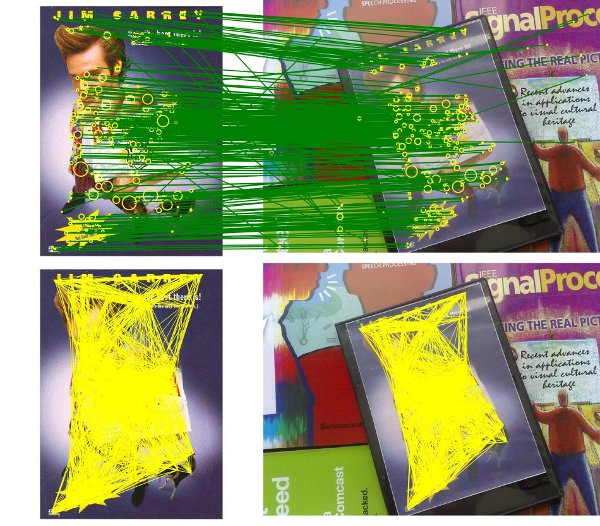# matching¶

## Metric customization¶

• L2 (used by default):
$d(x,y):=\| x-y \|_2$
• i.e L1 for binary descriptor (Hamming distance),
$d(x,y) = \sum(x \oplus y)$
• user customized distance (L1, ...).

## Image domain, correspondences filtering (KVLD)¶

When used with descriptors found putative matches can be filtered thanks to different filters:

• Symmetric distance (Left-Right check).
• Keep only mutal correspondences: a match is kept if it is the same in the A->B and B->A order.
$\lbrace (P^i_A,P^j_B): j = \underset{k} {\mathrm{argmin}} ~ d(\text{desc}(P^i_A), \text{desc}(P^k_B)), i = \underset{k} \min ~ d(\text{desc}(P^k_A), \text{desc}(P^j_B)) \rbrace$
• “Nearest Neighbor Distance Ratio” distance check can be performed to remove repetitive elements.
• As many nearest points have been asked we can measure the similarity between the N-nearest neighbor. If the ratio of distance is inferior to a threshold \delta the match is kept else it is rejected (since the risk of confusion is higher). It allows to avoid ambiguous correspondences. \delta is often chosen between 0.6 and 0.8.
$\lbrace (P^i_A,P^j_B): j = \underset{k} {\mathrm{argmin}} ~ d(\text{desc}(P^i_A), \text{desc}(P^k_B)) < \delta \underset{k\neq j} \min ~ d(\text{desc}(P^i_A), \text{desc}(P^k_B)) \rbrace$
• K-VLD Filter (K-Virtual Line Descriptor) [KVLD12]
• A virtual segment (a segment/line between two points) is kept if at least one of it’s supporting point is linked to K virtual segments. It produces a coherent photometric graph of the features from the set of points A and B. Below: Top (SIFT putative matches found by NNS), Bottom: K-VLD coherent matches.